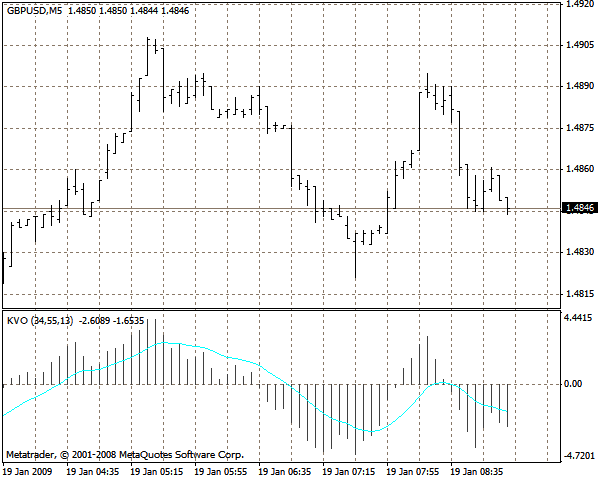# Klinger Oscillator KVO – indicator for MetaTrader 4

• A+
category：MT4 INDICATORS

This oscillator based on turnovers has been developed by Stephen Klinger.

The oscillator is calculated in seven steps:

1. The average typical price is calculated - (H+L+C)/3
2. If the current typical price is greater than the average typical price of the previous bar, the turnover of the current bar gets a "plus" sign
3. If the current typical price is less than the average typical price of the previous bar, the turnover of the current bar gets a "minus" sign
4. A 34-period EMA of the turnover obtained in steps 2 or 3 is calculated
5. A 55-period EMA of the turnover obtained in steps 2 or 3 is calculated
6. The 34-period EMA is subtracted from the 55-period EMA; the result is plotted on the chart as a histogram
7. The EMA of the difference obtained in step 6 is calculated and plotted on the chart